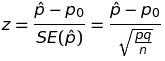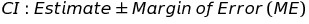## One-proportion z test in R

For quite a while, I’ve been confused by the behavior of the prop.test function in R: 1) the p-values always seem to be slightly off, and 2) the confidence intervals never seem to be symmetric around my estimate of the population proportion. Today, I decided I was going to figure out what was up… and whether I should trust my own analytical calculations for this test or what I get in R. I started by checking R Bloggers to see if anyone had explored this problem, but I only found one post about the two proportion z-test, and found none focusing on the one proportion z-test.

Here’s the essence of the test: you observe x subjects out of a sample size of n observations. Perhaps you just want to create a confidence interval to get a sense of where the true population proportion lies. Or maybe, you’d like to test the null hypothesis that the true population proportion is some value p0, against the alternative that it is really greater than, less than, or not equal to that value.

To do this, you can use the normal approximation to the binomial distribution to calculate a test statistic z. The estimated population proportion, p-hat, is just x/n. You have to find the standard error (SE) of your estimate as well to get the value of z:The confidence interval is constructed from the estimated population proportion, and the margin of error, which is determined by the standard error of the estimate and a scaling factor (z*) that establishes the width of the confidence interval:Here’s an example. Let’s say I know that nationally, 90% of home buyers would select a home with energy efficient features, even paying a premium of 2-3%, to reduce their utility costs long term. As the hypothetical president of an engineering firm that installs these energy efficient features, I want to know if less than 90% of buyers in my local area feel this way (because if so, I might want to launch an education campaign to help my business). I randomly sample 360 potential buyers and find that 312 of them would indeed select a home with energy efficient features.

I want to test my sample against the null hypothesis that the true population proportion is 0.90, with the alternative that the proportion is less than 0.90. I use a estimated population proportion (p-hat) of 312/360=0.867, and using the equations above, find that my test statistic z turns out to be -2.108, with a corresponding p-value of 0.0175. I reject the null hypothesis that the true population proportion is 0.90 in favor of the alternative, and start making plans to launch my education program.

(I don’t get the same values when I use prop.test. But later in this post, I’ll show you why I shouldn’t have expected that in the first place!!)

I wrote an R function to automate my z test (and also, to double check my calculations):

```z.test <- function(x,n,p=NULL,conf.level=0.95,alternative="less") {
ts.z <- NULL
cint <- NULL
p.val <- NULL
phat <- x/n
qhat <- 1 - phat
# If you have p0 from the population or H0, use it.
# Otherwise, use phat and qhat to find SE.phat:
if(length(p) > 0) {
q <- 1-p
SE.phat <- sqrt((p*q)/n)
ts.z <- (phat - p)/SE.phat
p.val <- pnorm(ts.z)
if(alternative=="two.sided") {
p.val <- p.val * 2
}
if(alternative=="greater") {
p.val <- 1 - p.val
}
} else {
# If all you have is your sample, use phat to find
# SE.phat, and don't run the hypothesis test:
SE.phat <- sqrt((phat*qhat)/n)
}
cint <- phat + c(
-1*((qnorm(((1 - conf.level)/2) + conf.level))*SE.phat),
((qnorm(((1 - conf.level)/2) + conf.level))*SE.phat) )
return(list(estimate=phat,ts.z=ts.z,p.val=p.val,cint=cint))
}```

When I run it on my example above, I get what I expect:

```> z.test(312,360,p=0.9)
\$estimate
 0.867

\$ts.z
 -2.11

\$p.val
 0.0175

\$cint
 0.836 0.898

```

But when I run prop.test, this isn’t what I see at all!

```> prop.test(312,360,p=0.9)

1-sample proportions test with continuity correction

data: 312 out of 360, null probability 0.9
X-squared = 4.08, df = 1, p-value = 0.04335
alternative hypothesis: true p is not equal to 0.9
95 percent confidence interval:
0.826 0.899
sample estimates:
p
0.867

```

Why are there differences? Here’s what I found out:

• The prop.test function doesn’t even do a z test. It does a Chi-square test, based on there being one categorical variable with two states (success and failure)! Which I should have noticed immediately from the line that starts with “X-squared” 🙂
• The Yates continuity correction, which adjusts for the differences that come from using a normal approximation to the binomial distribution, is also automatically applied. This removes 0.5/n from the lower limit of the confidence interval, and adds 0.5/n to the upper limit.
• Most notably, I was really disturbed to see that the confidence interval given by prop.test wasn’t even centered around the sample estimate, p-hat. Oh no! But again, this is no concern, since prop.test uses the Wilson score interval to construct the confidence interval. This results in an asymmetric, but presumably more accurate (with respect to the real population) confidence interval.

Moral of the story? There are many ways to test an observed proportion against a standard, target, or recommended value. If you’re doing research, and especially if you’re using R, be sure to know what’s going on under the hood before you report your results.

(Other moral? Try binom.test.)

•Additionally, you can also carry out the exact binomial test using the binom.test() fubction. This is what I typically use.

• Pingback: Distilled News | Data Analytics & R

•First, don’t use prop.test(), which uses a *terrible* large-sample approximation (and so does your z.test()). Instead, use binom.test(), which was written for this purpose.

Second, confidence intervals for binomial proportions should *not* be symmetric. E.g. for an esimated proportion of .99, the lower limit should obviously be further away from .99 than the upper limit (otherwise the confidence interval would be too narrow).

Third, actually *don’t* use binom.test(), as the p-value and the confidence interval need not be consistent (the confidence interval is not made by inverting the test). Instead, use the exactci package. See http://cran.r-project.org/web/packages/exactci/vignettes/exactci.pdf and http://journal.r-project.org/archive/2010-1/RJournal_2010-1_Fay.pdf (the latter is about the two-sample case, but also has an example for the one-sample case).This is exactly the kind of recommendation I’d hoped for. Thank you!! Going to start using exactci later today.

• James SuleimanGood work. If you are only interested in the p-value, wouldn’t prop.test(312, 360, p=.9, alternative=”less”, correct=FALSE) suffice? The above statement doesn’t apply Yates’ correction for continuity.Yep. My primary goal was pedagogical. Don’t want students blindly applying any of the methods… which they do all the time. In the default state (for the CI), prop.test applies the corrections, and I was bummed that none of them were noticing the numbers were different!

• Bruce DudekIf you apply the continuity correction to your Z expression (add .5/360 to your numerator since it is a negative deviation), you obtain a Z value of -2.02034. If you square this, you obtain 4.08179. It is not coincidental that the squared Z equals the Chi-square value, since a 1df chi-square distribution is a squared std normal Z. So, in fact, the prop.test function does do a “Z test”. It is just scaled as a Chi square.Ha! Hadn’t noticed that before. Now it seems so obvious. Thanks.

• Will LoweIt gets worse. All the standard frequentist intervals have bad coverage (and not even uniformly bad either). The Wald interval is simply the most egregious example. See Brown, Cai, and Dasgupta’s 2001 Statistical Science article (http://www-stat.wharton.upenn.edu/~tcai/paper/html/Binomial-StatSci.html) for the scary graphs. Ironically, this paper also notes you can do rather well on converage just going Bayesian and using a Jeffreys prior.Yikes… those graphs ARE scary. This paper is refreshingly well written too… thanks so much for posting!!

•There are multiple lessons for anyone who wishes to delve into this particular problem.

The first point to get out of the way is that logically Wald-type approaches (“standard error” etc) must be wrong! It is perfectly possible to observe a rate p = 0 or 1, but it is impossible to apply a symmetric interval about such a range without overshoot. In fact, the Wald-approaches are premised on a population value P not the observed value p. The problem is that >95% of people who write about intervals, or plot graphs, seem to blithely use such measures centred on their observations. E.B. Wilson, who pointed out the fallacy of this reasoning back in 1927, must be turning in his grave.

Like Brown et al (and Newcombe before them), I was sufficiently bothered about this issue to apply some brute computing power to empirically evaluating intervals. See this blog post and paper.

First, you can use the Newton-Raphson method of iterative approximation to invert the Binomial formula, log-likelihood, Gaussian, Yates’ etc, to obtain a confidence interval on p the hard way. This is how the Exact CI method works – inverting the Binomial. This allows you to plot the differences between the actual interval calculations for some reasonably representative and ‘risky’ cases (low frequency and skewed cases).

Second, one can choose whichever method you prefer for evaluating intervals – I decided to go for an exhaustive computation of all possible tests within a given framework and simply count the discrepancies (false positives, false negatives) against a baseline test (Fisher and Binomial). Instead of focusing on hypothetical saw-teeth as Brown et al do, this pulls out where the trade-offs empirically appear.Yes!! Yes, yes, YES!! So I’m at the culmination of writing an inquiry-based intro stats textbook, and I decided that as a part of this process, I would review every single approach and method I used and really get to know them deeply. I’ve been horrified as I delve into the particularities of Wald-type approaches (and actually, confidence intervals on proportions in general). Why do we still teach that garbage? Why do we think students should not be introduced to things like “coverage probabilities” in their intro classes? (Although I like your false +/false – approach better, because yeah, those plots invite questions like “why are they sawtoothy? why are they symmetric?” As a consequence of my eyes being opened, I’ve decided to change the way I teach my intro students, and consequently write up what I think are very valuable lessons that you and others have articulated so well. THANK YOU 🙂

I’m also a Six Sigma Black Belt, and horrified that concepts like this *aren’t* even touched on in training or the examination process. I have now started to freak out about all the decisions, research results, process improvements (etc.) that have been done so woefully inaccurately.

In any case, thanks for your post. It warms my heart to have heard from someone I have recently started to admire from afar 🙂

•Hi Nicole

Thanks for the code snippet

I think you need to replace your first line of p value calculation

p.val <- pnorm(ts.z)

With e.g.

p.val <- min(pnorm(ts.z),1-pnorm(ts.z))

R calculates the left tail, so when your score is positive and you have two tailed alternative, you get a p value of more than one :O)